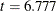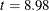The SYSLIN Procedure

Restricting Parameter Estimates

You can impose restrictions on the parameter estimates with RESTRICT and SRESTRICT statements. The RESTRICT statement imposes linear restrictions on parameters in the equation specified by the preceding MODEL statement. The SRESTRICT statement imposes linear restrictions that relate parameters in different models.

To impose restrictions involving parameters in different equations, use the SRESTRICT statement. Specify the parameters in the linear hypothesis as model-label.regressor-name. (If the MODEL statement does not have a label, you can use the dependent variable name as the label for the model, provided the dependent variable uniquely labels the model.)

Tests for the significance of the restrictions are printed when RESTRICT or SRESTRICT statements are used. You can label RESTRICT and SRESTRICT statements to identify the restrictions in the output.

The RESTRICT statement in the following example restricts the price coefficient in the demand equation to equal 0.015. The SRESTRICT statement restricts the estimate of the income coefficient in the demand equation to be 0.01 times the estimate of the unit cost coefficient in the supply equation.

```proc syslin data=in 3sls;
endogenous  p;
instruments y u s;
demand: model q = p y s;
peq015: restrict p = .015;
supply: model q = p u;
yeq01u: srestrict demand.y = .01 * supply.u;
run;
```

The restricted estimation results are shown in Figure 29.9.

Figure 29.9: Restricted Estimates

The SYSLIN Procedure
Three-Stage Least Squares Estimation

Model DEMAND q Quantity

Parameter Estimates
Variable DF Parameter
Estimate
Standard
Error
t Value Pr > |t| Variable
Label
Intercept 1 -0.46584 0.053307 -8.74 <.0001 Intercept
p 1 0.015000 0 . . Price
y 1 -0.00679 0.002357 -2.88 0.0056 Income
s 1 0.325589 0.009872 32.98 <.0001 Price of Substitutes
RESTRICT -1 50.59353 7.464988 6.78 <.0001 PEQ015

Model SUPPLY q Quantity

Parameter Estimates
Variable DF Parameter
Estimate
Standard
Error
t Value Pr > |t| Variable
Label
Intercept 1 -1.31894 0.477633 -2.76 0.0077 Intercept
p 1 1.291718 0.059101 21.86 <.0001 Price
u 1 -0.67887 0.235679 -2.88 0.0056 Unit Cost

Parameter Estimates
Variable DF Parameter
Estimate
Standard
Error
t Value Pr > |t| Variable
Label
RESTRICT -1 342.3605 38.12094 8.98 <.0001 YEQ01U

The standard error for P in the demand equation is 0, since the value of the P coefficient was specified by the RESTRICT statement and not estimated from the data. The "Parameter Estimates" table for the demand equation contains an additional row for the restriction specified by the RESTRICT statement. The parameter estimate for the restriction is the value of the Lagrange multiplier used to impose the restriction. The restriction is highly significant (), which means that the data are not consistent with the restriction, and the model does not fit as well with the restriction imposed. See the section RESTRICT Statement for details.

Following the "Parameter Estimates" table for the supply equation, the results for the cross model restrictions are printed. This shows that the restriction specified by the SRESTRICT statement is not consistent with the data (). See the section SRESTRICT Statement for details.